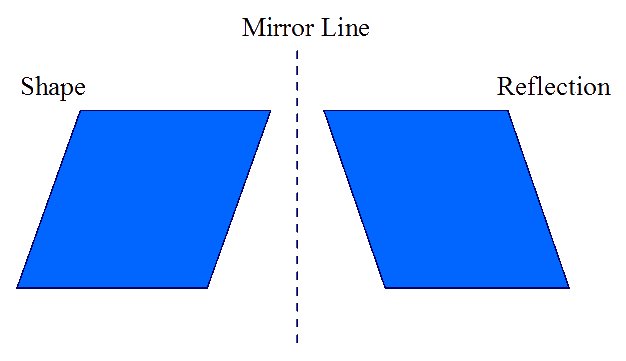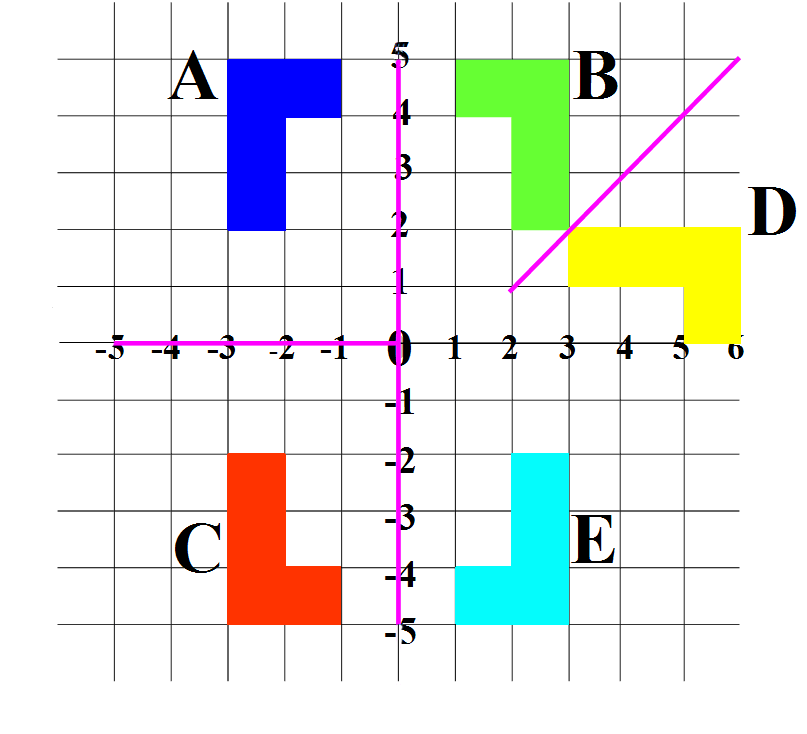# Transformation: Reflection

## Reflection is the image that you would see if you looked at a shape in a mirror. Your child needs to be able to identify the reflection of a shape along a line called a mirror line and be able to draw the reflection of a shape along a mirror line.

### The reflection has the same shape as the original, it is the same size and is the same distance away from the mirror line.In the diagram below, shape A is reflected along a vertical mirror line which coincides with the Y axis of a grid. The reflected image is shown as B. You can see that each vertex (corner) of shape B is the same distance from the mirror line as the corresponding vertex of shape A.Shape B is an exact mirror image of shape A. In school, children use small mirrors to help them to visualise this concept by holding the mirror on the mirror line.

### Mirror lines can be vertical, horizontal or diagonal.Shape C is a reflection of shape A along the mirror line of the X axis. this line has the coordinates (-5,0) to (0,0). At every point along this line the Y coordinate is zero - this line can therefore be called ... Y = 0

Shape E is a reflection of shape C along the mirror line of the Y axis. this line has the coordinates (0,5) to (0,-5). At every point along this line the X coordinate is zero - this line can therefore be called ... X = 0

Shape B is a reflection of shape A along the mirror line of the Y axis. this line has the coordinates (0,5) to (0,-5). At every point along this line the X coordinate is zero - this line can therefore be called ... X = 0

Shape D is a reflection of shape B along the diagonal mirror line with coordinates (2,1) to (6,5)

It is important to remember that each vertex of the reflected shape is the same distance from the mirror line as the corresponding vertex of the original shape.

The best way to draw a reflected shape is to plot the corresponding vertices as points
on the grid, ensuring that they are the same distance from the mirror line,
then join the points to form the shape.The best way to learn this is by practise. Download and print some quartered squared paper  from the 11plusforparents Printable Resources page, draw on some shapes and have your child reflect them using different mirror lines.

Practice working with Reflections with these worksheets from Primary Resources